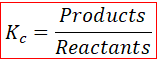# Problem: Consider the following reaction at equilibrium: CO(g) + Cl2(g) ⇌ COCl2(g)Predict whether the reaction will shift left, shift right, or remain unchanged if Cl2 is added to the reaction mixture.

###### FREE Expert Solution

According to Le Chatelier’s Principle if a system (chemical reaction) is at equilibrium and we disturb it, then the system will readjust to maintain its equilibrium state.

The equilibrium expression can be written as,CO (g) + Cl(g)  COCl(g)

Chemical equilibrium is a state in which the rate of forward reaction equals the backward reaction

• The equilibrium constant K is a number that is equal to the ratio of products to reactants at a given temperature.

99% (378 ratings)###### Problem Details

Consider the following reaction at equilibrium: CO(g) + Cl2(g) ⇌ COCl2(g)

Predict whether the reaction will shift left, shift right, or remain unchanged if Cl2 is added to the reaction mixture.